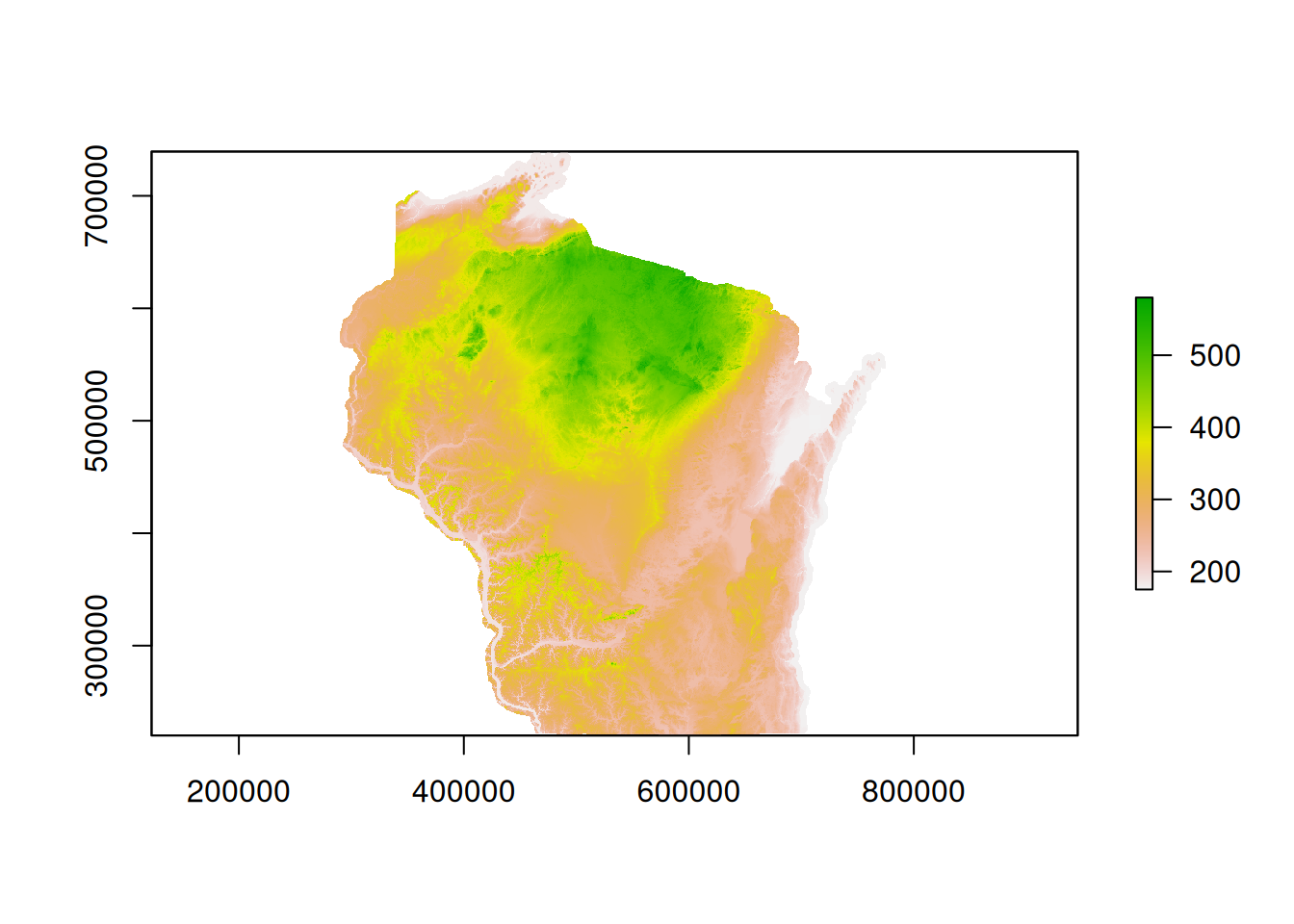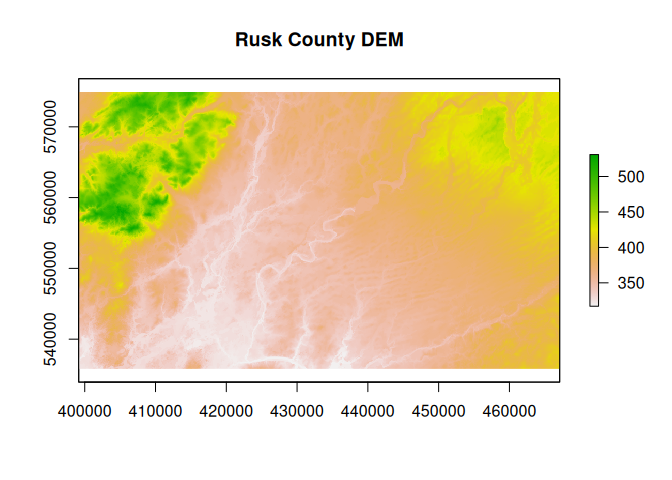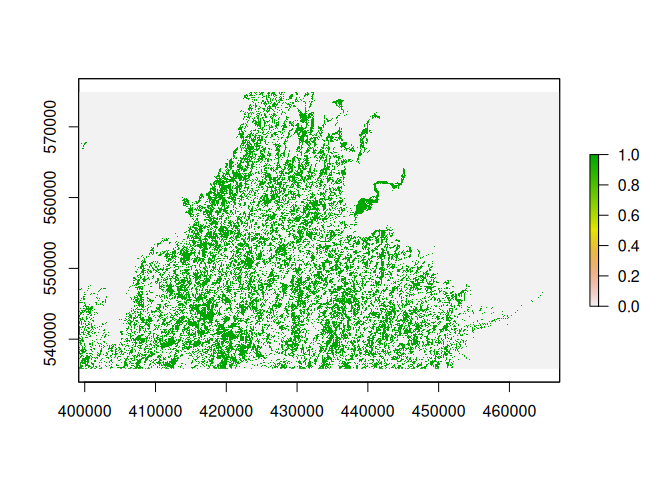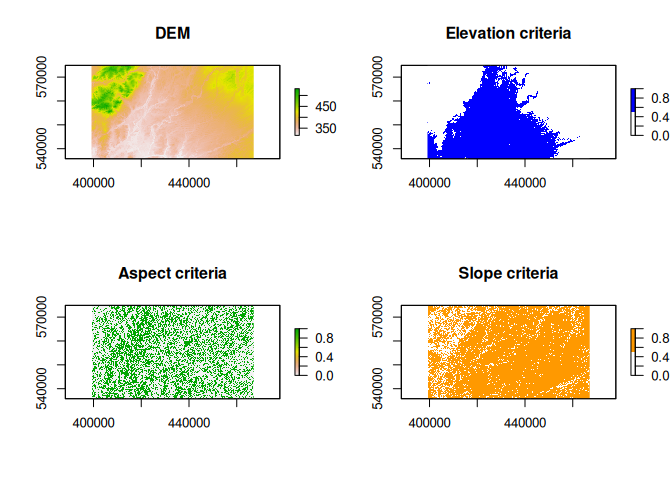# Site Suitability/Terrain Analysis in R

In my GIS 2 class, I have students complete a simple site suitability analysis using Model Builder and ArcGIS. I’d like to have them do this through scripting, but at the time of assignment I think it’s a bit above their comfort level. I created this post as a demonstration of how to do site suitability/terrain analysis in R.

Suppose a client wants to build a cabin in Rusk County, Wisconsin and requests that it be situated situated

1. Below 1200 ft.
2. On a slope of less than 3 degrees
3. Facing northeast, east, or southeast (angle between 22.5 and 157.5 degrees)

First, we will load packages then download and unzip the DEM data.

``````library(here)
library(tigris)
library(raster)
library(dplyr)
library(sf)
library(measurements)
library(leaflet)

## download dem and use the "here" package to set a "relative" working directory
destfile = here("data/DEM_30m.zip"))
## unzip dem data
unzip(here("data/DEM_30m.zip"), exdir = here("data"))

wi.dem <- raster(here("data/DEM_30m/demgw930/"))``````
``plot(wi.dem)``Next, we will download county data for Wisconsin, extract just Rusk County, and transform the CRS into that of the DEM.

``````## use sf instead of sp, use cartographic boundary files since they look nicer
rusk.co <- counties("55", class = "sf", cb = TRUE, progress_bar = FALSE) %>%
filter(NAME == "Rusk") %>%
st_transform(., crs(wi.dem))``````

After this, we can crop the DEM to the county boundary since everything outside of Rusk County is irrelevant.

``````## crop dem to country boundaries
rusk.dem <- crop(wi.dem, rusk.co)

plot(rusk.dem, main = "Rusk County DEM")``````Next, we are ready to implement terrain analysis functions and complete the binary site suitability analysis. We can simply use the `<` operator to get all cells under 1200 ft. Then, we can “chain” the other functions together using the `&` operator. Here, we can use the `terrain` function and specify the `opt` argument.

``````rusk.suit <-
## elevation; under 1200 ft, convert to m (units of the dem)
rusk.dem < conv_unit(1200, "ft", "m") &
## aspect; between 22.5 and 157.5 degrees
terrain(rusk.dem, opt = "aspect", unit = "degrees") > 22.5 &
terrain(rusk.dem, opt = "aspect", unit = "degrees") < 157.5 &
## slope; less than 3 degrees
terrain(rusk.dem, opt = "slope", unit = "degrees") < 3

plot(rusk.suit)``````Of course, the individual criteria may be of interest on their own. If that is the case, then we can create a function that completes each operation within its own `plot` function and displays each criteria individually.

``````plot_criteria <- function(elev, asp.min, asp.max, slope) {
## get plot parameters so we can reset them after plotting is done
opar <- par()

## set plot parameters
par(mfrow = c(2,2))

## plot each step
plot(rusk.dem, main = "DEM")
plot(rusk.dem < conv_unit(elev, "ft", "m"), main = "Elevation criteria",
col = c("#ffffff", "#0000ff"))
plot(terrain(rusk.dem, opt = "aspect", unit = "degrees") > asp.min &
terrain(rusk.dem, opt = "aspect", unit = "degrees") < asp.max, main = "Aspect criteria")
plot(terrain(rusk.dem, opt = "slope", unit = "degrees") < slope, main = "Slope criteria",
col = c("#ffffff", "#ff9900"))

## reset plot parameters
par(opar)
}

plot_criteria(1200, 22.5, 157.5, 3)``````It would also be nice to visualize the final result with some context. To do this, we can use a `leaflet` basemap.

``````leaflet(width = "100%") %>%